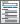Home  /  Products  /  Features  /  Graphics

## Publication-quality graphics

Many graph styles

Graphic features

• Added-variable plots (partial-regression leverage plots)
• Component-plus-residual plots (partial residual plots)
• Augmented component-plus-residual plots (augmented partial-residual plots)
• Leverage-versus-squared residual plots
• Residual-versus-fitted plots
• Residual-versus-predictor plots (independent variable plots)

Distributional diagnostic plotsMargins plots, profile plots, and interaction plotsSmoothing

Item response theory

Multivariate graphs

Nonparametric regression conditional mean plotsQuality control• Unicode characters
• Bold and italic
• Serif and sans serif
• Monospace and proportional
• Greek letters
• Mathematical symbols
• Superscripts and subscripts
• Remove
• Move
• Modify
• Record and apply edits to other graphs

Meta-analysis

Lasso

Bayesian graphsSurvival plots

Time-series graphs

Panel-data line plots• Graphs by panel
• Overlaid panels
• Automated and customizable
• Comparative — multiple lines, subgraphs, or graphs

Causal inference/treatment effects

ROC analysis